Courses

# JEE Main Mathematics Mock - 5

## 25 Questions MCQ Test Mock Test Series for JEE Main & Advanced 2022 | JEE Main Mathematics Mock - 5

Description
This mock test of JEE Main Mathematics Mock - 5 for JEE helps you for every JEE entrance exam. This contains 25 Multiple Choice Questions for JEE JEE Main Mathematics Mock - 5 (mcq) to study with solutions a complete question bank. The solved questions answers in this JEE Main Mathematics Mock - 5 quiz give you a good mix of easy questions and tough questions. JEE students definitely take this JEE Main Mathematics Mock - 5 exercise for a better result in the exam. You can find other JEE Main Mathematics Mock - 5 extra questions, long questions & short questions for JEE on EduRev as well by searching above.
QUESTION: 1

Solution:
QUESTION: 2

Solution:
QUESTION: 3

### [(1+cosθ+i sinθ)/(i+sinθ+i cosθ)]4 = cos nθ + isin nθ, then n=

Solution:
QUESTION: 4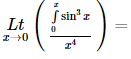Solution:
QUESTION: 5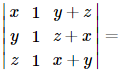Solution:
QUESTION: 6

The differential equation which represents the family of plane curves y=exp. (cx) is

Solution: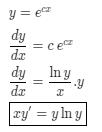QUESTION: 7

If the domain of function f(x) = x2 - 6x + 7 is (-∞, ∞), then the range of function is :

Solution:
QUESTION: 8

In the following question, a Statement-1 is given followed by a corresponding Statement-2 just below it. Read the statements carefully and mark the correct answer-
Let a, b, c, p, q be real numbers. Suppose α,β are the roots of the equation x2 + 2px + q = 0 and a, 1/β are the roots of the equation ax2 + 2bx + c = 0, where β2 ∉ {-1, 0, 1}
Statement-1:
(p2-q)(b2-ac)≥0
Statement-2:
b≠pa or c≠qa

Solution: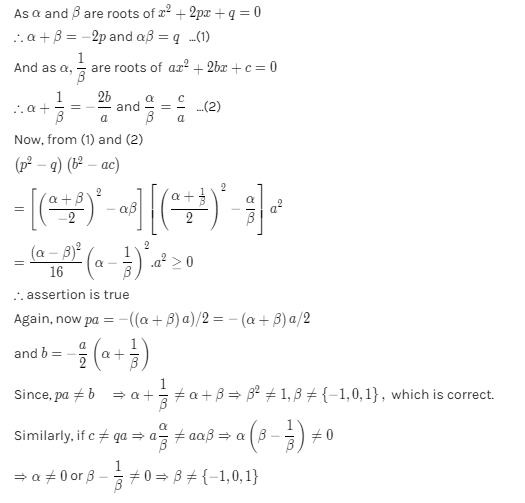QUESTION: 9

The angle between the pair of tangents drawn from the point (1,2) to the ellipse 3x2 + 2y2= 5 is

Solution:
QUESTION: 10

(d/dx)[cos(1-x2)2]=

Solution:
QUESTION: 11

In the following question, a Statement of Assertion (A) is given followed by a corresponding Reason (R) just below it. Read the Statements carefully and mark the correct answer-
Assertion(A): ex , loge x are two functions such that each is the image of the other with respect to the line x = y
Reason (R): The inverse of every bijective function is symmetric about the line x = y

Solution:
QUESTION: 12

A square tank of capacity 250 cubic m has to be dug out. The cost of land is Rs 50 per sq.m. The cost of digging increases with the depth and for the whole tank is 400 (depth)2 rupees. The dimensions of the tank for the least total cost are

Solution:
QUESTION: 13

The same roots of 3-4 i are

Solution:
QUESTION: 14

Everybody in a room shakes hands with every body else. The total number of hand shakes is 66. Then the number of persons in the room is

Solution:

There are n people in the room
n(n - 1)/2 = 66
n2 - n = 132
n2 - n - 132 = 0
n2 - 12n + 11n - 132 = 0
n(n - 12) + 11(n - 12) = 0
(n + 11) (n - 12) = 0
n = 12, - 11
Hence total no of persons = 12

QUESTION: 15

If the sum of the squares of the roots of x2 + px - 3 = 0 is 10, then the values of p =

Solution: Sum of the roots of equation :- α + β = - b/aProducts of the roots of equation :- αβ = c/a squaring on both side sum of roots of equation α^2 +β^2 +2αβ = b^2/a^2 put values in above equation 10 + 2(-3) =b^2 b = +2 or b = -2
QUESTION: 16

Three identical dice are rolled. The probability that the same number will appear on each of them, is

Solution:
QUESTION: 17

If A is the single A.M. between two numbers a and b and S is the sum of n A.M.'s between them, then S/A depends upon

Solution:
QUESTION: 18
If the two lines of regression are 5x + 3y = 55 and 7x + y = 45, then the correlation coefficient between x and y is
Solution:
QUESTION: 19

M telegrams are distributed at random over N communication channels (N > M). The probability of the event
A = {not more than one telegram will be sent over any channel} is

Solution:

The total no. of ways of distributing M telegrams over N channels = NM
The number of ways of choosing M channels out of N to send one telegram over each channel = NCM
∴ Total no. of ways to send M telegrams over each channel = NCM . M!
∴ Required probubility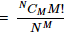QUESTION: 20

The equation of a line passing through (-a,0) and form a triangle of area 'T' with coordinates axes, is

Solution:
*Answer can only contain numeric values
QUESTION: 21

If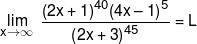then value of L/128 is :-

Solution: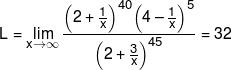*Answer can only contain numeric values
QUESTION: 22

If the function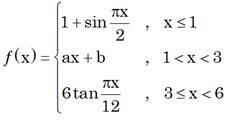is continuous in the interval (–∞, 6) then value of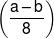is

Solution: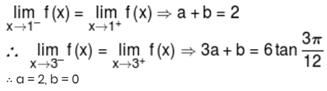*Answer can only contain numeric values
QUESTION: 23

Value of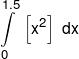is equals to
(where [.] denotes greatest integer function)

Solution: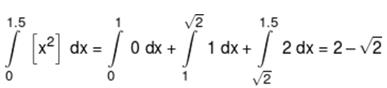*Answer can only contain numeric values
QUESTION: 24

If the area bounded by curve x + |y| = 1 and the y-axis is k, then k/4 is equals to

Solution: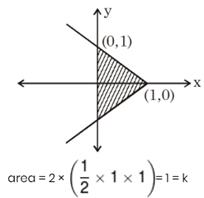*Answer can only contain numeric values
QUESTION: 25

If value of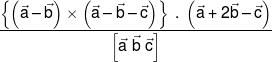is k then k/4 is

Solution: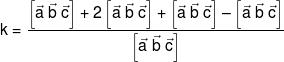k = 3